# Measurement of lowering of vapour pressure

MEASUREMENT OF LOWERING OF VAPOUR PRESSURE

## (1) Barometric Method

Raoult measured the individual vapour pressure of a liquid and then the solution by this method.

– He introduced the liquid or the solution into Toricellian vacuum of a barometer tube and measured the depression of the mercury level.

– This method is neither practicable nor accurate as the lowering of vapour pressure is too small.

## (2) Manometric Method

– The vapour pressure of a liquid or solution can be conveniently measured with the help of a manometer (see Fig).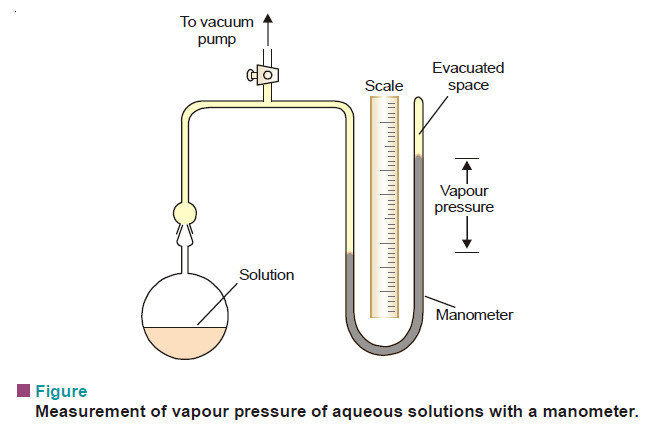– The bulb B is charged with the liquid or solution. The air in the connecting tube in then removed with a vacuum pump.

– When the stopcock is closed, the pressure inside is due only to the vapour evaporating from the solution or liquid.

– This method is generally used for aqueous solutions.

– The manometric liquid can be mercury or n-butyl phthalate which has low density and low volatility.

## (3) Ostwald and Walker’s Dynamic Method (Gas Saturation Method)

– In this method the relative lowering of vapour pressure can be determined straightway.

– The measurement of the individual vapour pressures of a solution and solvent is thus eliminated.

### Procedure

The apparatus used by Ostwald and Walker is shown in the following Fig.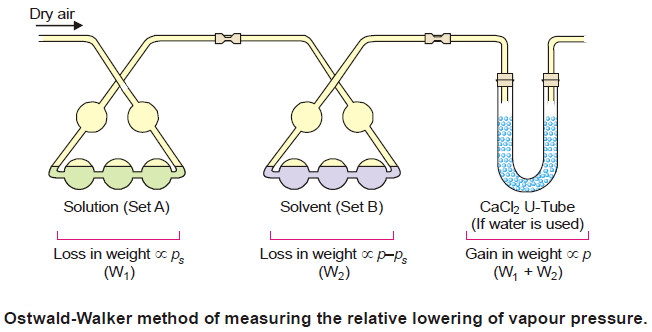– It consists of two sets of bulbs :

(a) Set (A) containing the solution

(b) Set (B) containing the solvent

– Each set is weighed separately.

– A slow stream of dry air is then drawn by suction pump through the two sets of bulbs.

– At the end of the operation, these sets are reweighed. From the loss of weight in each of the two sets, the lowering of vapour pressure is calculated.

– The temperature of the air, the solution and the solvent must be kept constant throughout.

### Calculations

– As the air bubbles through set (A) it is saturated up to the vapour pressure ps of solution and then up to vapour pressure p of solvent in set (B).

– Thus the amount of solvent taken up in set (A) is proportional to ps and the amount taken up in set (B) is proportional to (p – ps).

– If w1 and w2 be the loss of weight in set A and B respectively,

w1 ∝ ps       …(1)

w2 ∝ p – ps      …(2)

w1 + w2 ∝ ps + p – ps

∝ p      …(3)

Dividing (2) by (3), we can write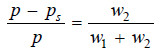(4)

– Knowing the loss of mass in set B (w2) and the total loss of mass in the two sets (w1 + w2), we can find the relative lowering of vapour pressure from equation (4).

– If water is the solvent used, a set of calcium chloride tubes (or a set of bulbs containing conc. H2SO4) is attached to the end of the apparatus to catch the escaping water vapour. Thus the gain in mass of the CaCl2-tubes is equal to (w1 + w2), the total loss of mass in sets (A) and (B).

## SOLVED PROBLEM

Problem (1): A current of dry air was passed through a solution of 2.64 g of benzoic acid in 30.0 g of ether (C2H5OC2H5) and then through pure ether. The loss in weight of the solution was 0.645 g and the ether 0.0345 g. What is the molecular mass of benzoic acid ?

solution

According to the theory of Ostwald-Walker method,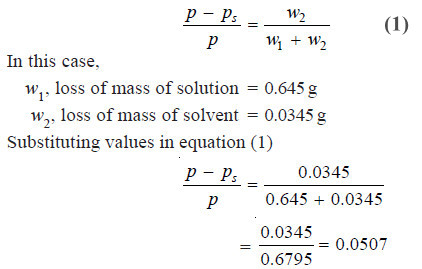From Raoult’s Law, we have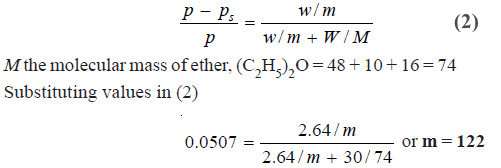Hence, m, the molecular mass of benzoic acid = 122

Problem (2): A stream of dry air was passed through a bulb containing a solution of 7.50 g of an aromatic compound in 75.0 g of water and through another globe containing pure water. The loss in mass in the first globe was 2.810 g and in the second globe it was 0.054 g. Calculate the molecular mass of the aromatic compound. (Mol mass of water = 18)

solution

According to the theory of Ostwald-Walker method,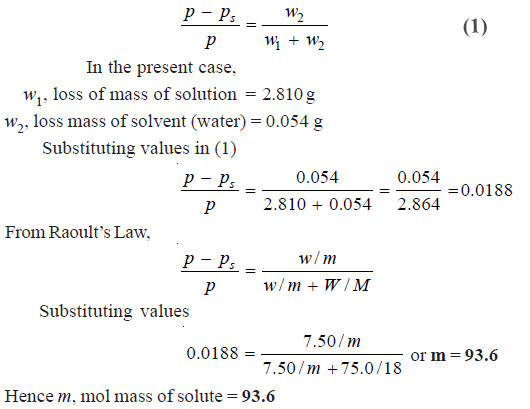Problem (3): In an experiment air was drawn successively through a solution of sugar (38.89 g per 100 g water) and distilled water, and then through anhydrous calcium chloride. It was found that the water lost was 0.0921 g and calcium chloride tubes gained 5.163 g. Find the molecular mass of the sugar. (Mol mass of H2O = 18)

solution

According to the theory of Ostwald-Walker method,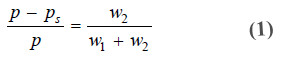In this case,
w2, the loss of mass of water = 0.0921 g
(w1 + w2), the total loss of solution and solvent = 5.163 g

Substituting values in equation (1)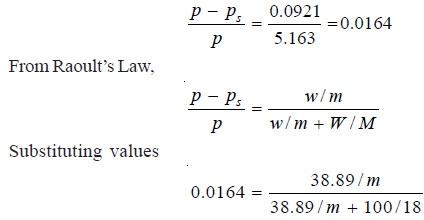Hence m, mol mass of sugar = 385